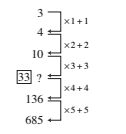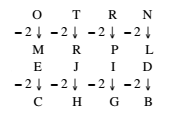# RRB ALP 2018 Practice Test Papers | Reasoning Questions (Day-28)

Dear Aspirants, Here we have given the Important RRB ALP & Technicians Exam 2018 Practice Test Papers. Candidates those who are preparing for RRB ALP 2018 can practice these Reasoning Questions to get more confidence to Crack RRB 2018 Examination.

[WpProQuiz 1955]

Click “Start Quiz” to attend these Questions and view Solutions

1. BFJ: R:: OAF:?

(A) P

(B) Q

(C) V

(D) W

1. XPT: JHL:: BDL:?

(A) FAB

(B) FXB

(C) FBC

(D) FDA

1. Find out the missing term in the following series— 3, 4, 10,?, 136, 685

(A) 39

(B) 36

(C) 33

(D) 42

1. In some code language, OTRN is written as MRPL. How can EJID be written in the same language?

(A) BGHC

(B) GIKF

(C) CHGB

(D) DBGH

1. Natural: Artificial:: Spontaneous:?

(A) Calculated

(B) Imprompt

(C) Instinctive

(D) Free of all

1. Ram is the father of Sushil and Kiran. Sushil is the son of Ram but Kiran is not the son of Ram. How is Kiran related to Ram?

(A) Son-in-law

(B) Niece

(C) Mother

(D) Daughter

1. Which of the words given below cannot be formed from the given word?

SIGNIFICANT

(A) NASCENT

(B) INFANT

(C) INSIGNIA

(D) GIANT

1. Jyoti remembers that her brother’s birthday is after 17th but before 21st Feb., while her brother remembers that his birthday is after 19th but before 24th Feb. On which date is his birthday?

(A) 22 Feb.

(B) 21 Feb.

(C) 18 Feb.

(D) 20 Feb.

1. Raghav is 7th from the top and 29th from the bottom in the class. How many students are in the class?

(A) 36

(B) 34

(C) 33

(D) 35

1. A, B, C, D and E are five rivers. A is shorter than B but longer than E. C is the longest and D is a little shorter than B and a little longer than A. Which is the shortest river?

(A) A

(B) B

(C) C

(D) E

B F J: R:: O A F:?

⇒ (2 + 6 + 10): 18:: (15 + 1 + 6):?

∴? = 22 × 18/ 18 = 22 = V

X P T: J H L:: B D L:?

⇒ (24 + 16 + 20): (10 + 8 + 12) :: (2 + 4 + 12):?

∴? = 30 × 18/ 60 = 9 = (6 + 1 + 2) = F A BAs ‘Artificial is opposite of ‘Natural’. In the same way ‘Calculated’ is opposite of ‘Spontaneous’.

Kiran is the child of Ram but not son. Therefore, Kiran will be daughter of Ram.

E is not present in the given word.

After 17th but before 21st means 18, 19 and 20 while after 19th but before 24th means 20, 21, 22 and 23. Since among these days only 20th is common. Therefore, his birthday is on 20th.

7+29-1=35

According to the decreasing in length, they are as follow— C B D A E

RRB ALP 2018 Practice Test Papers | Reasoning Questions (Day-1)

RRB ALP 2018 Practice Test Papers | Reasoning Questions (Day-2)

RRB ALP 2018 Practice Test Papers | Reasoning Questions (Day-3)

RRB ALP 2018 Practice Test Papers | Reasoning Questions (Day-4)

RRB ALP 2018 Practice Test Papers | Reasoning Questions (Day-5)

RRB ALP 2018 Practice Test Papers | Reasoning Questions (Day-6)

RRB ALP 2018 Practice Test Papers | Reasoning Questions (Day-7)

RRB ALP 2018 Practice Test Papers | Reasoning Questions (Day-8)

RRB ALP 2018 Practice Test Papers | Reasoning Questions (Day-9)

RRB ALP 2018 Practice Test Papers | Reasoning Questions (Day-10)

RRB ALP 2018 Practice Test Papers | Reasoning Questions (Day-11)

RRB ALP 2018 Practice Test Papers | Reasoning Questions (Day-12)

RRB ALP 2018 Practice Test Papers | Reasoning Questions (Day-13)

RRB ALP 2018 Practice Test Papers | Reasoning Questions (Day-14)

RRB ALP 2018 Practice Test Papers | Reasoning Questions (Day-15)

RRB ALP 2018 Practice Test Papers | Reasoning Questions (Day-16)

RRB ALP 2018 Practice Test Papers | Reasoning Questions (Day-17)

RRB ALP 2018 Practice Test Papers | Reasoning Questions (Day-18)

RRB ALP 2018 Practice Test Papers | Reasoning Questions (Day-19)

RRB ALP 2018 Practice Test Papers | Reasoning Questions (Day-20)

RRB ALP 2018 Practice Test Papers | Reasoning Questions (Day-21)

RRB ALP 2018 Practice Test Papers | Reasoning Questions (Day-22)

RRB ALP 2018 Practice Test Papers | Reasoning Questions (Day-23)

RRB ALP 2018 Practice Test Papers | Reasoning Questions (Day-24)

RRB ALP 2018 Practice Test Papers | Reasoning Questions (Day-25)

RRB ALP 2018 Practice Test Papers | Reasoning Questions (Day-26)

RRB ALP 2018 Practice Test Papers | Reasoning Questions (Day-27)